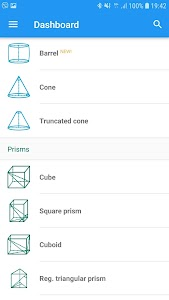Geometry v2.27

Free

Geometry / ScreenshotsGeometry / Description

"Geometry" is advanced application for solving geometry problems. There is a complete solution delivered for each issue to satisfy every teacher or student.

The application solves every algebraic problem including those with:
- fractions
- roots
- powers
you can also use parentheses decimal numbers and Pi number.

This application is able to do calculation on the following figures:
- Triangles: equilateral triangle right triangle isosceles triangle 30-60-90
- Quadrilaterals: square rectangle rhombus parallelogram trapezoid right trapezoid isosceles trapezoid kite
- Polygons: regular pentagon regular hexagon
- circle ellipse annulus and annulus sector
- Solids of revolution: sphere cylinder cone spherical sector spherical cap
- Prisms: cube square prism cuboid
- Pyramids: regular tetrahedron
- Others: Pythagorean theorem trigonometry
- PRO version: square pyramid triangular pyramid triangular prism regular triangular prism Thales' theorem truncated cone regular octagon regular dodecagon hexagonal prism hexagonal pyramid pentagonal prism barrel law of sines law of cosines spherical wedge spherical lune spherical segment spherical zone

An advanced validation of data entry allows you to find the errors quickly and corrects them for you immediately.

"Geometry" will calculate all the parameters of the figure if you enter the necessary data. The order of data entry depends on you!

- Do you want to calculate a side of the square? No problem. "Geometry" will do it for you.
- Do you have an angle and a side of the right triangle? Perfect. Other values can be calculated.

None of your geometry tasks will be a problem now with "Geometry". This application has very advanced powerful and easy - to - use interface.

Additionally it contains all the useful formulas which you will need to solve geometry tasks. But thats not enough! You do not have to figure it out how did you get a result. This application not only gives you the solution it also shows you all the formulas which had been used. Pythagorean Theorem sines and cosines are no longer a problem.

Geometry / What's New in v2.27

2.27
- Dark theme

2.26
- Fixes

2.24
- New language: svenska

2.21
- Kite, Truncated cone, Regular triangular prism
-- Additional diagrams in the solution

2.20
- Trapezoid, Right trapezoid, Isosceles trapezoid
-- Additional diagrams in the solution